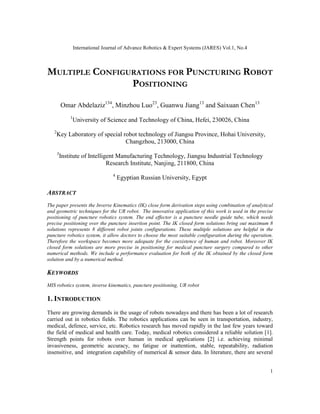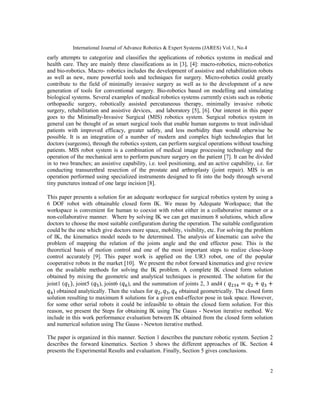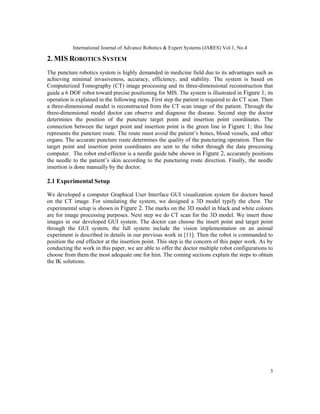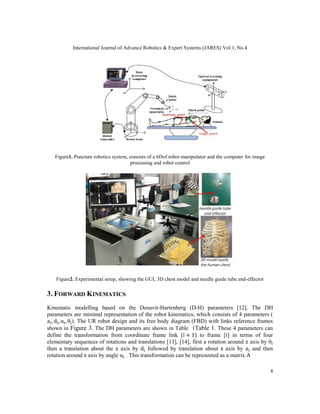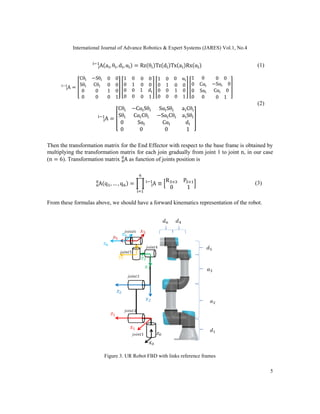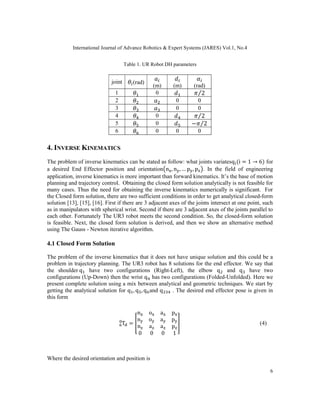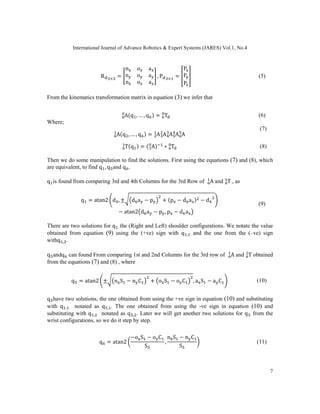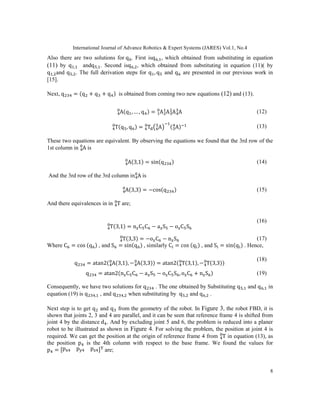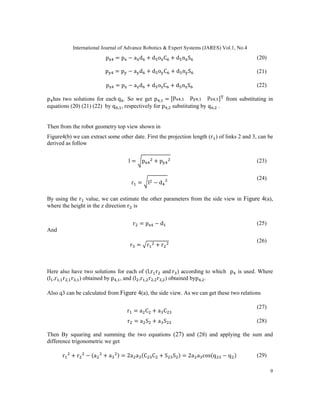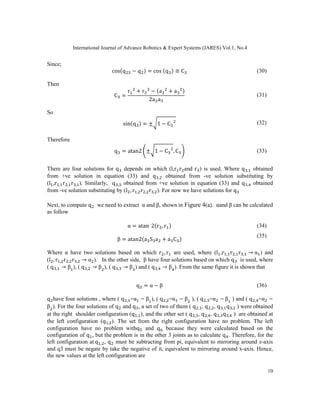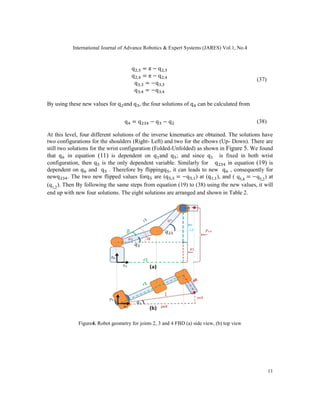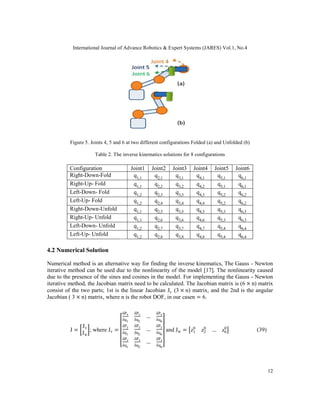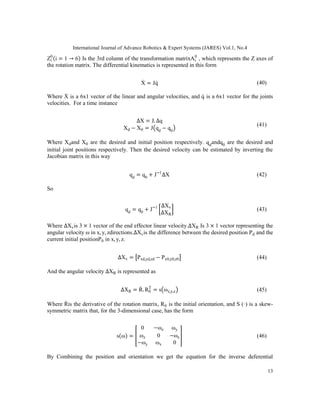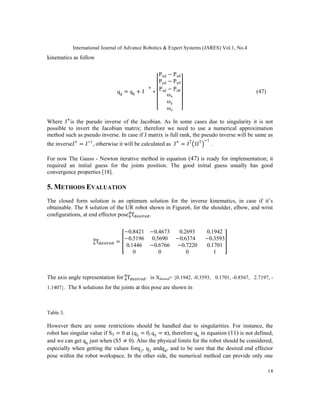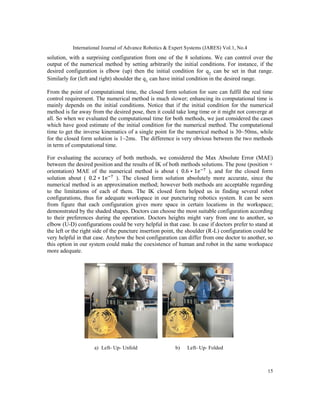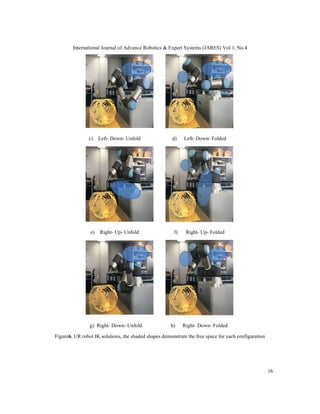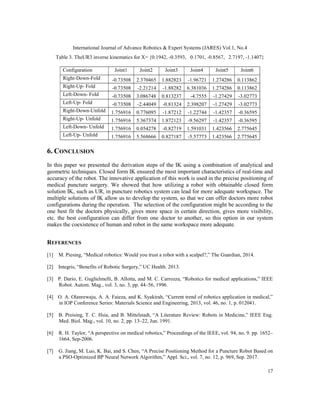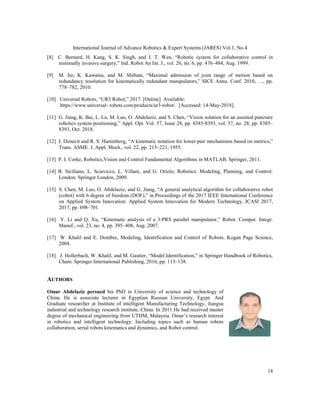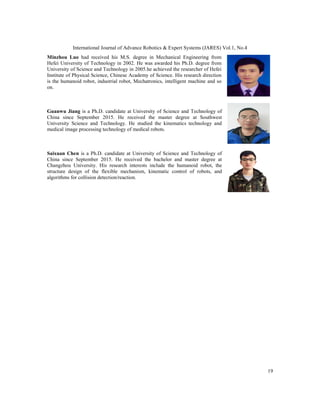Próximo SlideShare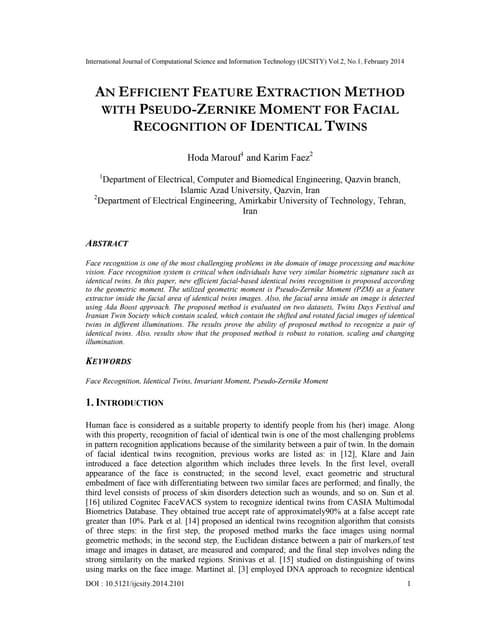An efficient feature extraction method with pseudo zernike moment for facial ...
Cargando en ... 3
1 de 19

### MULTIPLE CONFIGURATIONS FOR PUNCTURING ROBOT POSITIONING

1. International Journal of Advance Robotics & Expert Systems (JARES) Vol.1, No.4 1 MULTIPLE CONFIGURATIONS FOR PUNCTURING ROBOT POSITIONING Omar Abdelaziz134 , Minzhou Luo23 , Guanwu Jiang13 and Saixuan Chen13 1 University of Science and Technology of China, Hefei, 230026, China 2 Key Laboratory of special robot technology of Jiangsu Province, Hohai University, Changzhou, 213000, China 3 Institute of Intelligent Manufacturing Technology, Jiangsu Industrial Technology Research Institute, Nanjing, 211800, China 4 Egyptian Russian University, Egypt ABSTRACT The paper presents the Inverse Kinematics (IK) close form derivation steps using combination of analytical and geometric techniques for the UR robot. The innovative application of this work is used in the precise positioning of puncture robotics system. The end effector is a puncture needle guide tube, which needs precise positioning over the puncture insertion point. The IK closed form solutions bring out maximum 8 solutions represents 8 different robot joints configurations. These multiple solutions are helpful in the puncture robotics system, it allow doctors to choose the most suitable configuration during the operation. Therefore the workspace becomes more adequate for the coexistence of human and robot. Moreover IK closed form solutions are more precise in positioning for medical puncture surgery compared to other numerical methods. We include a performance evaluation for both of the IK obtained by the closed form solution and by a numerical method. KEYWORDS MIS robotics system, inverse kinematics, puncture positioning, UR robot 1. INTRODUCTION There are growing demands in the usage of robots nowadays and there has been a lot of research carried out in robotics fields. The robotics applications can be seen in transportation, industry, medical, defence, service, etc. Robotics research has moved rapidly in the last few years toward the field of medical and health care. Today, medical robotics considered a reliable solution . Strength points for robots over human in medical applications  i.e. achieving minimal invasiveness, geometric accuracy, no fatigue or inattention, stable, repeatability, radiation insensitive, and integration capability of numerical & sensor data. In literature, there are several
2. International Journal of Advance Robotics & Expert Systems (JARES) Vol.1, No.4 2 early attempts to categorize and classifies the applications of robotics systems in medical and health care. They are mainly three classifications as in , : macro-robotics, micro-robotics and bio-robotics. Macro- robotics includes the development of assistive and rehabilitation robots as well as new, more powerful tools and techniques for surgery. Micro-robotics could greatly contribute to the field of minimally invasive surgery as well as to the development of a new generation of tools for conventional surgery. Bio-robotics based on modelling and simulating biological systems. Several examples of medical robotics systems currently exists such as robotic orthopaedic surgery, robotically assisted percutaneous therapy, minimally invasive robotic surgery, rehabilitation and assistive devices, and laboratory , . Our interest in this paper goes to the Minimally-Invasive Surgical (MIS) robotics system. Surgical robotics system in general can be thought of as smart surgical tools that enable human surgeons to treat individual patients with improved efficacy, greater safety, and less morbidity than would otherwise be possible. It is an integration of a number of modern and complex high technologies that let doctors (surgeons), through the robotics system, can perform surgical operations without touching patients. MIS robot system is a combination of medical image processing technology and the operation of the mechanical arm to perform puncture surgery on the patient . It can be divided in to two branches; an assistive capability, i.e. tool positioning, and an active capability, i.e. for conducting transurethral resection of the prostate and arthroplasty (joint repair). MIS is an operation performed using specialized instruments designed to fit into the body through several tiny punctures instead of one large incision . This paper presents a solution for an adequate workspace for surgical robotics system by using a 6 DOF robot with obtainable closed form IK. We mean by Adequate Workspace; that the workspace is convenient for human to coexist with robot either in a collaborative manner or a non-collaborative manner. Where by solving IK we can get maximum 8 solutions, which allow doctors to choose the most suitable configuration during the operation. The suitable configuration could be the one which give doctors more space, mobility, visibility, etc. For solving the problem of IK, the kinematics model needs to be determined. The analysis of kinematic can solve the problem of mapping the relation of the joints angle and the end effector pose. This is the theoretical basis of motion control and one of the most important steps to realize close-loop control accurately . This paper work is applied on the UR3 robot, one of the popular cooperative robots in the market . We present the robot forward kinematics and give review on the available methods for solving the IK problem. A complete IK closed form solution obtained by mixing the geometric and analytical techniques is presented. The solution for the joint1 ( ), joint5 ( ), joint6 ( ), and the summation of joints 2, 3 and4 ( = + + ) obtained analytically. Then the values for , , obtained geometrically. The closed form solution resulting to maximum 8 solutions for a given end-effector pose in task space. However, for some other serial robots it could be infeasible to obtain the closed form solution. For this reason, we present the Steps for obtaining IK using The Gauss - Newton iterative method. We include in this work performance evaluation between IK obtained from the closed form solution and numerical solution using The Gauss - Newton iterative method. The paper is organized in this manner. Section 1 describes the puncture robotic system. Section 2 describes the forward kinematics. Section 3 shows the different approaches of IK. Section 4 presents the Experimental Results and evaluation. Finally, Section 5 gives conclusions.
3. International Journal of Advance Robotics & Expert Systems (JARES) Vol.1, No.4 3 2. MIS ROBOTICS SYSTEM The puncture robotics system is highly demanded in medicine field due to its advantages such as achieving minimal invasiveness, accuracy, efficiency, and stability. The system is based on Computerized Tomography (CT) image processing and its three-dimensional reconstruction that guide a 6 DOF robot toward precise positioning for MIS. The system is illustrated in Figure 1; its operation is explained in the following steps. First step the patient is required to do CT scan. Then a three-dimensional model is reconstructed from the CT scan image of the patient. Through the three-dimensional model doctor can observe and diagnose the disease. Second step the doctor determines the position of the puncture target point and insertion point coordinates. The connection between the target point and insertion point is the green line in Figure 1; this line represents the puncture route. The route must avoid the patient’s bones, blood vessels, and other organs. The accurate puncture route determines the quality of the puncturing operation. Then the target point and insertion point coordinates are sent to the robot through the data processing computer. The robot end-effector is a needle guide tube shown in Figure 2, accurately positions the needle to the patient’s skin according to the puncturing route direction. Finally, the needle insertion is done manually by the doctor. 2.1 Experimental Setup We developed a computer Graphical User Interface GUI visualization system for doctors based on the CT image. For simulating the system, we designed a 3D model typify the chest. The experimental setup is shown in Figure 2. The marks on the 3D model in black and white colours are for image processing purposes. Next step we do CT scan for the 3D model. We insert these images in our developed GUI system. The doctor can choose the insert point and target point through the GUI system, the full system include the vision implementation on an animal experiment is described in details in our previous work in . Then the robot is commanded to position the end effector at the insertion point. This step is the concern of this paper work. As by conducting the work in this paper, we are able to offer the doctor multiple robot configurations to choose from them the most adequate one for him. The coming sections explain the steps to obtain the IK solutions.
4. International Journal of Adv Figure1. Puncture robotics system, consists of a 6Dof robot manipulator and the computer for image Figure2. Experimental setup, showing the GUI, 3D chest model and ne 3. FORWARD KINEMATICS Kinematic modelling based on the Denavit parameters are minimal representation of the robot kinematics, which consists of 4 parameters ( a , d , α , θ ). The UR robot design and its free body diagram (FBD) with links reference frames shown in Figure 3. The DH parameters are shown in define the transformation from coordinat elementary sequences of rotations and translations then a translation about the z rotation around x axis by angle International Journal of Advance Robotics & Expert Systems (JARES) Vol.1, No.4 Puncture robotics system, consists of a 6Dof robot manipulator and the computer for image processing and robot control Experimental setup, showing the GUI, 3D chest model and needle guide tube end INEMATICS Kinematic modelling based on the Denavit-Hartenberg (D-H) parameters  parameters are minimal representation of the robot kinematics, which consists of 4 parameters ( ). The UR robot design and its free body diagram (FBD) with links reference frames . The DH parameters are shown in Table 1Table 1. These 4 parameters can define the transformation from coordinate frame link i + 1 to frame i in terms of four elementary sequences of rotations and translations , , first a rotation around axis by d followed by translation about x axis by axis by angle α . This transformation can be represented as a matrix pert Systems (JARES) Vol.1, No.4 4 Puncture robotics system, consists of a 6Dof robot manipulator and the computer for image edle guide tube end-effector . The DH parameters are minimal representation of the robot kinematics, which consists of 4 parameters ( ). The UR robot design and its free body diagram (FBD) with links reference frames . These 4 parameters can in terms of four , first a rotation around z axis by θ axis by a and then . This transformation can be represented as a matrix A
5. International Journal of Adv A(a , θ , d A = Cθ −Sθ 0 0 Sθ Cθ 0 0 0 0 0 0 1 0 0 1 A = C S Then the transformation matrix for the End Effector with respect to the base frame is obtained by multiplying the transformation matrix for each join gradually from joint 1 to joint (n = 6). Transformation matrix A(q , … , From these formulas above, we should have a forward kinematics representation of the robot. Figure 3 International Journal of Advance Robotics & Expert Systems (JARES) Vol.1, No.4 d , α ) = Rz(θ )Tz(d )Tx(a )Rx(α ) 1 0 0 0 0 1 0 0 0 0 0 0 1 d 0 1 1 0 0 α 0 1 0 0 0 0 0 0 1 0 0 1 1 0 0 0 0 Cα −Sα 0 0 0 Sα 0 Cα 0 0 1 Cθ −Cα Sθ Sα Sθ a Cθ Sθ Cα Cθ −Sα Cθ a Sθ 0 Sα Cα d 0 0 0 1 Then the transformation matrix for the End Effector with respect to the base frame is obtained by multiplying the transformation matrix for each join gradually from joint 1 to joint ). Transformation matrix A as function of joints position is q ) = A ≡ R × P × 0 1 From these formulas above, we should have a forward kinematics representation of the robot. 3. UR Robot FBD with links reference frames pert Systems (JARES) Vol.1, No.4 5 (1) (2) Then the transformation matrix for the End Effector with respect to the base frame is obtained by multiplying the transformation matrix for each join gradually from joint 1 to joint n, in our case (3) From these formulas above, we should have a forward kinematics representation of the robot.
6. International Journal of Advance Robotics & Expert Systems (JARES) Vol.1, No.4 6 Table 1. UR Robot DH parameters joint (rad) (m) (m) (rad) 1 0 2⁄ 2 0 0 3 0 0 4 0 2⁄ 5 0 − 2⁄ 6 0 0 0 4. INVERSE KINEMATICS The problem of inverse kinematics can be stated as follow: what joints variatesq (i = 1 → 6) for a desired End Effector position and orientation n , n , … p , p . In the field of engineering application, inverse kinematics is more important than forward kinematics. It’s the base of motion planning and trajectory control. Obtaining the closed form solution analytically is not feasible for many cases. Thus the need for obtaining the inverse kinematics numerically is significant. For the Closed form solution, there are two sufficient conditions in order to get analytical closed-form solution , , . First if there are 3 adjacent axes of the joints intersect at one point, such as in manipulators with spherical wrist. Second if there are 3 adjacent axes of the joints parallel to each other. Fortunately The UR3 robot meets the second condition. So, the closed-form solution is feasible. Next, the closed form solution is derived, and then we show an alternative method using The Gauss - Newton iterative algorithm. 4.1 Closed Form Solution The problem of the inverse kinematics that it does not have unique solution and this could be a problem in trajectory planning. The UR3 robot has 8 solutions for the end effector. We say that the shoulder q have two configurations (Right-Left), the elbow q and q have two configurations (Up-Down) then the wrist q has two configurations (Folded-Unfolded). Here we present complete solution using a mix between analytical and geometric techniques. We start by getting the analytical solution for q , q , q and q . The desired end effector pose is given in this form T = n o a p n o a p n a a p 0 0 0 1 (4) Where the desired orientation and position is
7. International Journal of Advance Robotics & Expert Systems (JARES) Vol.1, No.4 7 R × = n o a n o a n o a , P × = P P P (5) From the kinematics transformation matrix in equation (3) we infer that A(q , … , q ) = T (6) Where; A(q , … , q ) = A A A A A (7) T(q ) = ( A) ∗ T (8) Then we do some manipulation to find the solutions. First using the equations (7) and (8), which are equivalent, to find q , q and q . q is found from comparing 3rd and 4th Columns for the 3rd Row of A and T , as q = atan2 d , ± d a − p + (p − d a ) − d − atan2 d a − p , p − d a (9) There are two solutions for q the (Right and Left) shoulder configurations. We notate the value obtained from equation (9) using the (+ve) sign with q , and the one from the (–ve) sign withq , . q andq can found From comparing 1st and 2nd Columns for the 3rd row of A and T obtained from the equations (7) and (8) , where q = atan2 ± n S − n C + o S − o C , a S − a C (10) q have two solutions, the one obtained from using the +ve sign in equation (10) and substituting with q , notated as q , . The one obtained from using the -ve sign in equation (10) and substituting with q , notated as q , . Later we will get another two solutions for q from the wrist configurations, so we do it step by step. q = atan2 −o S − o C S , n S − n C S (11)
8. International Journal of Advance Robotics & Expert Systems (JARES) Vol.1, No.4 8 Also there are two solutions for q . First isq , , which obtained from substituting in equation (11) by q , andq , . Second isq , , which obtained from substituting in equation (11)( by q , and q , . The full derivation steps for q , q and q are presented in our previous work in . Next, q = (q + q + q ) is obtained from coming two new equations (12) and (13). A(q , … , q ) = A A A A (12) T(q , q ) = T A ( A) (13) These two equations are equivalent. By observing the equations we found that the 3rd row of the 1st column in A is A(3,1) = sin(q ) (14) And the 3rd row of the 3rd column in A is A(3,3) = −cos(q ) (15) And there equivalences in in T are; T(3,1) = n C C − a S − o C S (16) T(3,3) = −o C − n S (17) Where C = cos (q ) , and S = sin(q ) , similarly C = cos (q ) , and S = sin(q ) . Hence, q = atan2( A(3,1), − A(3,3)) = atan2( T(3,1), − T(3,3)) (18) q = atan2(n C C − a S − o C S ,  o C + n S  ) (19) Consequently, we have two solutions for q . The one obtained by Substituting q , and q , in equation (19) is q , , and q , when substituting by q , and q , . Next step is to get q and q from the geometry of the robot. In Figure 3, the robot FBD, it is shown that joints 2, 3 and 4 are parallel, and it can be seen that reference frame 4 is shifted from joint 4 by the distance d . And by excluding joint 5 and 6, the problem is reduced into a planer robot to be illustrated as shown in Figure 4. For solving the problem, the position at joint 4 is required. We can get the position at the origin of reference frame 4 from T in equation (13), as the position p is the 4th column with respect to the base frame. We found the values for p = p p p are;
9. International Journal of Advance Robotics & Expert Systems (JARES) Vol.1, No.4 9 p = p − a d + d o C + d n S (20) p = p − a d + d o C + d n S (21) p = p − a d + d o C + d n S (22) p has two solutions for each q . So we get p , = p , p , p , from substituting in equations (20) (21) (22) by q , , respectively for p , substituting by q , . Then from the robot geometry top view shown in Figure4(b) we can extract some other date. First the projection length (r ) of links 2 and 3, can be derived as follow l = p + p (23) r = l − d (24) By using the r value, we can estimate the other parameters from the side view in Figure 4(a), where the height in the z direction r is r = p − d (25) And r = r + r (26) Here also have two solutions for each of (l,r r and r ) according to which p is used. Where (l ,r , r , r , ) obtained by p , , and (l ,r , r , r , ) obtained byp , . Also q3 can be calculated from Figure 4(a), the side view. As we can get these two relations r = a C + a C (27) r = a S + a S (28) Then By squaring and summing the two equations (27) and (28) and applying the sum and difference trigonometric we get r + r − (a + a ) = 2a a (C C + S S ) = 2a a cos(q − q ) (29)
10. International Journal of Advance Robotics & Expert Systems (JARES) Vol.1, No.4 10 Since; cos(q − q ) = cos (q ) ≡ C (30) Then C = r + r − (a + a ) 2a a (31) So sin(q ) = ± 1 − C (32) Therefore q = atan2 ± 1 − C , C (33) There are four solutions for q depends on which (l,r r and r ) is used. Where q , obtained from +ve solution in equation (33) and q , obtained from -ve solution substituting by (l ,r , r , r , ). Similarly, q , obtained from +ve solution in equation (33) and q , obtained from -ve solution substituting by (l , r , r , r , ). For now we have solutions for q Next, to compute q we need to extract α and β, shown in Figure 4(a). αand β can be calculated as follow α = atan 2(r , r ) (34) β = atan2(a S a + a C ) (35) Where α have two solutions based on which r , r are used, where (l ,r , r , r , → α ) and (l , r , r , r , → α ). In the other side, β have four solutions based on which q is used, where ( q , → β ), ( q , → β ), ( q , → β ) and ( q , → β ). From the same figure it is shown that q = α − β (36) q have four solutions , where ( q , =α − β ), ( q , =α − β ), ( q , =α − β ) and ( q , =α − β ). For the four solutions of q and q , a set of two of them ( q , , q , , q , q , ) were obtained at the right shoulder configuration (q , ), and the other set ( q , , q , , q , q , ) are obtained at the left configuration (q , ). The set from the right configuration have no problem. The left configuration have no problem withq and q because they were calculated based on the configuration of q , but the problem is in the other 3 joints as to calculate q . Therefore, for the left configuration at q , , q must be subtracting from pi, equivalent to mirroring around z-axis and q3 must be negate by take the negative of it, equivalent to mirroring around x-axis. Hence, the new values at the left configuration are
11. International Journal of Adv By using these new values for q At this level, four different solutions of the inverse kinematics are obtained. The solutions have two configurations for the shoulders (Right still two solutions for the wrist configuration (Folded that q in equation (11) is dependent on configuration, then q is the only dependent on q and q . Therefore by flipping newq . The two new flipped values for (q1,2 ). Then By following the same steps from equation end up with new four solutions. The eight solutions are arranged and shown in Figure4. Robot geometry for joints 2, 3 and 4 FBD (a) side view, (b) top view International Journal of Advance Robotics & Expert Systems (JARES) Vol.1, No.4 q , = π − q , q , = π − q , q , = −q , q , = −q , q and q , the four solutions of q can be calculated from q = q − q − q At this level, four different solutions of the inverse kinematics are obtained. The solutions have two configurations for the shoulders (Right- Left) and two for the elbows (Up- Down). There are still two solutions for the wrist configuration (Folded-Unfolded) as shown in Figure is dependent on q and q ; and since q is fixed in both wrist is the only dependent variable. Similarly for q in equation . Therefore by flippingq , it can leads to new q , consequently for . The two new flipped values forq are (q , = −q , ) at (q , ), and ( q ). Then By following the same steps from equation (19) to (38) using the new values, it will end up with new four solutions. The eight solutions are arranged and shown in Table geometry for joints 2, 3 and 4 FBD (a) side view, (b) top view pert Systems (JARES) Vol.1, No.4 11 (37) can be calculated from (38) At this level, four different solutions of the inverse kinematics are obtained. The solutions have Down). There are Figure 5. We found is fixed in both wrist in equation (19) is , consequently for q5,4 = −q5,2 ) at using the new values, it will Table 2. geometry for joints 2, 3 and 4 FBD (a) side view, (b) top view
12. International Journal of Adv Figure 5. Joints 4, 5 and 6 at two different configurations Folded (a) and Unfolded (b) Table 2. The inverse kinematics solutions for 8 configurations Configuration Right-Down-Fold Right-Up- Fold Left-Down- Fold Left-Up- Fold Right-Down-Unfold Right-Up- Unfold Left-Down- Unfold Left-Up- Unfold 4.2 Numerical Solution Numerical method is an alternative way for finding the inverse kinematics, The Gauss iterative method can be used due to the nonlinearity of the model due to the presence of the sines and cosines in the model. For implementing the Gauss iterative method, the Jacobian matrix need to be calcul consist of the two parts; 1st is the linear Jacobian Jacobian ( 3 × n) matrix, where J = Jv Jw ; where Jv = International Journal of Advance Robotics & Expert Systems (JARES) Vol.1, No.4 . Joints 4, 5 and 6 at two different configurations Folded (a) and Unfolded (b) . The inverse kinematics solutions for 8 configurations Joint1 Joint2 Joint3 Joint4 Joint5 Joint6 q1,1 q2,1 q3,1 q4,1 q5,1 q q1,1 q2,2 q3,2 q4,2 q5,1 q q1,2 q2,3 q3,3 q4,3 q5,2 q q1,2 q2,4 q3,4 q4,4 q5,2 q q1,1 q2,5 q3,5 q4,5 q5,3 q q1,1 q2,6 q3,6 q4,6 q5,3 q q1,2 q2,7 q3,7 q4,7 q5,4 q q1,2 q2,8 q3,8 q4,8 q5,4 q Numerical method is an alternative way for finding the inverse kinematics, The Gauss iterative method can be used due to the nonlinearity of the model . The nonlinearity caused due to the presence of the sines and cosines in the model. For implementing the Gauss iterative method, the Jacobian matrix need to be calculated. The Jacobian matrix is ( consist of the two parts; 1st is the linear Jacobian Jv (3 × n) matrix, and the 2nd is the angular ) matrix, where n is the robot DOF, in our casen = 6. δPx δq1 δPx δq2 … δPx δq6 δPy δq1 δPy δq2 … δPy δq6 δPz δq1 δPz δq2 … δPz δq6 and Jw = z1 0 z2 0 … z6 0 pert Systems (JARES) Vol.1, No.4 12 . Joints 4, 5 and 6 at two different configurations Folded (a) and Unfolded (b) Joint6 q6,1 q6,1 q6,2 q6,2 q6,3 q6,3 q6,4 q6,4 Numerical method is an alternative way for finding the inverse kinematics, The Gauss - Newton . The nonlinearity caused due to the presence of the sines and cosines in the model. For implementing the Gauss - Newton ated. The Jacobian matrix is (6 × n) matrix ) matrix, and the 2nd is the angular (39)
13. International Journal of Advance Robotics & Expert Systems (JARES) Vol.1, No.4 13 Zi 0 (i = 1 → 6) Is the 3rd column of the transformation matrixAi 0 , which represents the Z axes of the rotation matrix. The differential kinematics is represented in this form X˙ = Jq˙ (40) Where X˙ is a 6x1 vector of the linear and angular velocities, and q˙ is a 6x1 vector for the joints velocities. For a time instance ∆X = J. ∆q Xd − X0 = J qd − q0 (41) Where Xdand X0 are the desired and initial position respectively. qd andq0 are the desired and initial joint positions respectively. Then the desired velocity can be estimated by inverting the Jacobian matrix in this way qd = q0 + J 1 ∆X (42) So qd = q0 + J 1 ∆Xv ∆XR (43) Where ∆Xvis 3 × 1 vector of the end effector linear velocity.∆XR Is 3 × 1 vector representing the angular velocity ω in x, y, zdirections.∆Xvis the difference between the desired position Pd and the current initial positionP0 in x, y, z. ∆Xv = Pxd,yd,zd − Px0,y0,z0 (44) And the angular velocity ∆XR is represented as ∆XR = R˙ . R0 T = s ωx,y,z (45) Where R˙ is the derivative of the rotation matrix, R0 is the initial orientation, and S (·) is a skew- symmetric matrix that, for the 3-dimensional case, has the form s(ω) = 0 −ωz ωy ωz 0 −ωx −ωy ωx 0 (46) By Combining the position and orientation we get the equation for the inverse deferential
14. International Journal of Advance Robotics & Expert Systems (JARES) Vol.1, No.4 14 kinematics as follow qd = q0 + J ∗ Pxd − Px0 Pyd − Py0 Pzd − Pz0 ωx ωy ωz (47) Where J is the pseudo inverse of the Jacobian. As In some cases due to singularity it is not possible to invert the Jacobian matrix; therefore we need to use a numerical approximation method such as pseudo inverse. In case if J matrix is full rank, the pseudo inverse will be same as the inverseJ = J 1 , otherwise it will be calculated as J = JT JJT 1 . For now The Gauss - Newton iterative method in equation (47) is ready for implementation; it required an initial guess for the joints position. The good initial guess usually has good convergence properties . 5. METHODS EVALUATION The closed form solution is an optimum solution for the inverse kinematics, in case if it’s obtainable. The 8 solution of the UR robot shown in Figure6, for the shoulder, elbow, and wrist configurations, at end effector pose T . T = −0.8421 −0.4673 0.2693 0.1942 −0.5196 0.5690 −0.6374 −0.3593 0.1446 −0.6766 −0.7220 0.1701 0 0 0 1 The axis angle representation for T . is Xdesired= {0.1942, -0.3593, 0.1701, -0.8567, 2.7197, - 1.1407}. The 8 solutions for the joints at this pose are shown in Table 3. However there are some restrictions should be handled due to singularities. For instance, the robot has singular value if S5 = 0 at (q5 = 0, q5 = π), therefore q6 in equation (11) is not defined, and we can get q6 just when (S5 ≠ 0). Also the physical limits for the robot should be considered, especially when getting the values forq2 , q3 andq4 , and to be sure that the desired end effector pose within the robot workspace. In the other side, the numerical method can provide only one